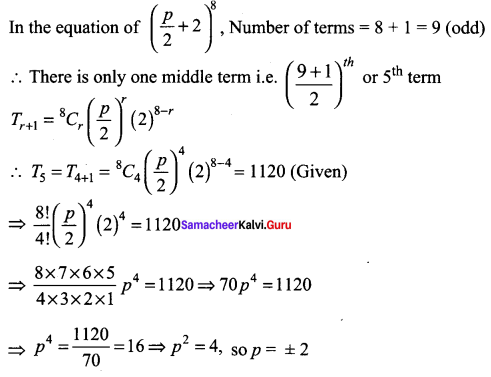## Tamilnadu Samacheer Kalvi 11th Maths Solutions Chapter 5 Binomial Theorem, Sequences and Series Ex 5.1

11th Maths Exercise 5.1 Question 1.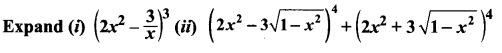Solution: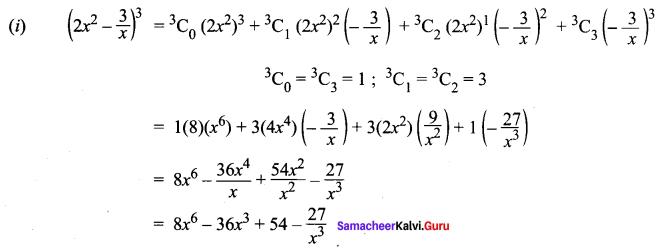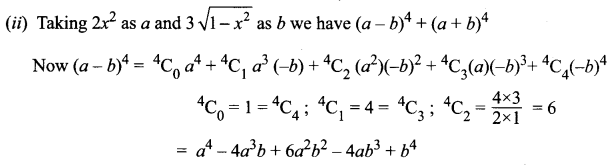Similarly (a + b)4 = a4 + 4a3b + 6a2b2 + 4ab3 + b4
∴ (a – b)4 + (a + b)4 = 2 [a4 + 6a2b2 + b4]
Substituting the value of a and b we get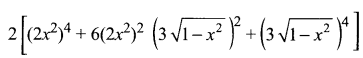= 2[16x8 + 6(4x4)(9(1 – x2)) + 81(1 – x2)2]
= 2[16x8 + 216x4(1 – x2) + 81(1 – x2)2]
= 2[16x8 + 216x4 – 216x6 + 81 + 81x4 – 162x2]
= 2[16x8 – 216x6 + 297x4 – 162x2 + 81]
= 32x8 – 432x6 + 594x4 – 324x2 + 162

11th Maths Exercise 5.1 Samacheer Kalvi Question 2.
Compute
(i) 1024
(ii) 994
(iii) 97
Solution:

(i) 1024 = (100 + 2)4 = (102 + 2)4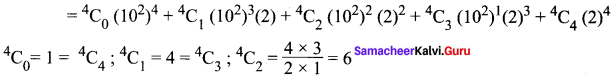= 1(108) + 4(106)(2) + 6(104)(4) + 4(102)(8) + 16
= 100000000 + 8000000 + 240000 + 3200 + 16
= 108243216

(ii) 994 = (100 – 1)4 = (102 – 1)4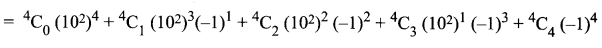= 1(108) + 4(106)(-1) + 6 (104)(1) + 4( 104)(-1) + (-1)4
= 100000000 – 4000000 + 60000 – 400 + 1
= 100060001 – 4000400 = 96059601

(iii) 97 = (10 – 1)7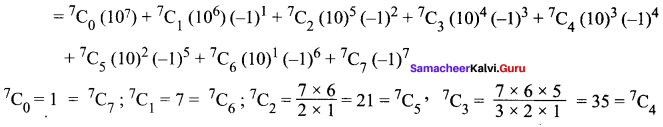= 1(10000000) + 7(1000000)(-1) + 21(100000)(1) + 35(10000)(-1) + 35(1000)(1) + 21(100)(-1) + 7(10)(1) + 1(-1)
= 10000000 – 7000000 + 2100000 – 350000 + 35000 – 2100 + 70 – 1
= 12135070 – 7352101 = 4782969

Sequences And Series Solutions Question 3.
Using binomial theorem, indicate which of the following two number is larger: (1.01)1000000, 10000.
Solution:
(1.01)1000000 = (1 + 0.01)1000000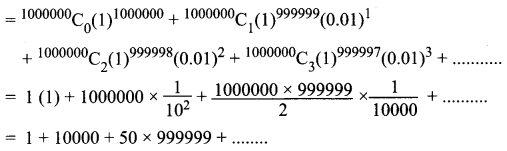which is > 10000
So (1.01)1000000 > 10000 (i.e.) (1.01)1000000 is larger

11 Maths Samacheer Kalvi Question 4.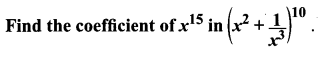Solution: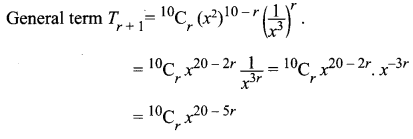To find coefficient of x15 we have to equate x power to 15
i.e. 20 – 5r = 15
20 – 15 = 5r ⇒ 5r = 5 ⇒ r = 5/5 = 1
So the coefficient of x15 is 10C1 = 10

Ex 5.1 Class 11 Question 5.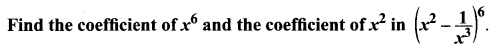Solution: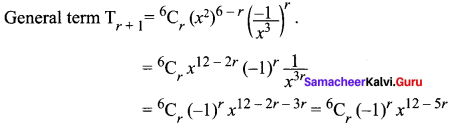To find coefficient of x6
12 – 5r = 6
12 – 6 = 5r ⇒ 5r = 6 ⇒ r = 6/5 which is not an integer.
∴ There is no term involving x6.
To find coefficient of x2
12 – 5r = 2
5r = 12 – 2 = 10 ⇒ r = 2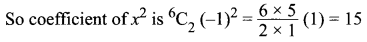Question 6.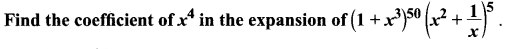Solution: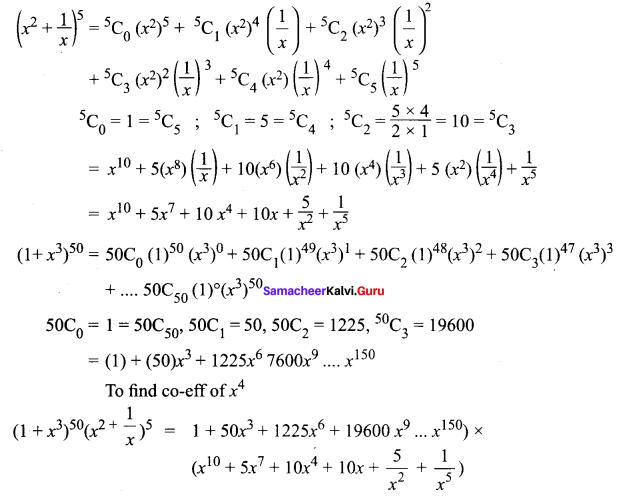when multiplying these terms, we get x4 terms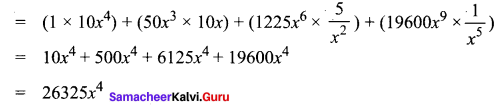∴ The co-eff of x4 is 26325

Ex 5.1 Class 11 Question 7.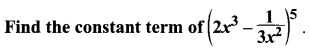Solution: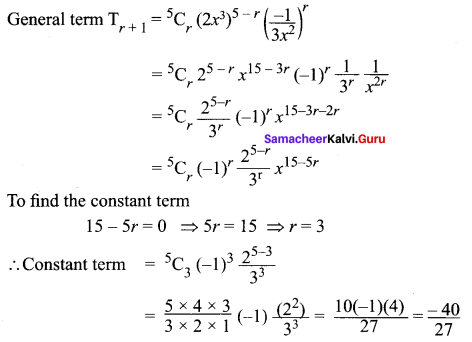Chapter 5 Class 11 Maths Question 8.
Find the last two digits of the number 3600.
Solution:
3600 = 32 × 300 = (9)300 = (10 – 1)300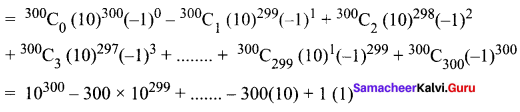All the terms except last term are ÷ by 100. So the last two digits will be 01.

Samacheer Kalvi 11th Maths Question 9.
If n is a positive integer, show that, 9n + 1 – 8n – 9 is always divisible by 64.
Solution: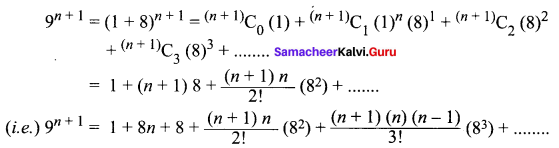∴ 9n + 1 – 8n – 9 = 64 [an integer]
⇒ 9n + 1 – 8n – 9 is divisible by 64

Class 11 Maths Binomial Theorem Question 10.
If n is an odd positive integer, prove that the coefficients of the middle terms in the expansion of (x + y)n are equal.
Solution:
Given n is odd. So let n = 2n + 1, where n is an integer.
The expansion (x + y)n has n + 1 terms.
= 2n + 1 + 1 = 2(n + 1) terms which is an even number.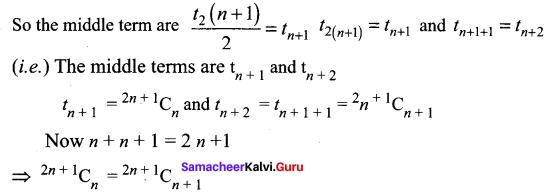⇒ The coefficient of the middle terms in (x + y)n are equal.

Class 11 Ex 5.1 Question 11.
If n is a positive integer and r is a non – negative integer, prove that the coefficients of xr and xn – r in the expansion of (1 + x)n are equal.
Solution: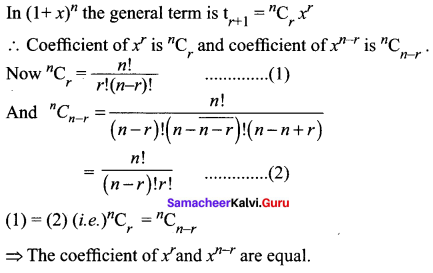Class 11 Maths Chapter 5 Exercise 5.1 Question 12.
If a and b are distinct Integers, prove that a – b is a factor of an – bn, whenever n is a positive integer. [Hint: write an = (a – b + b)n and expand]
Solution: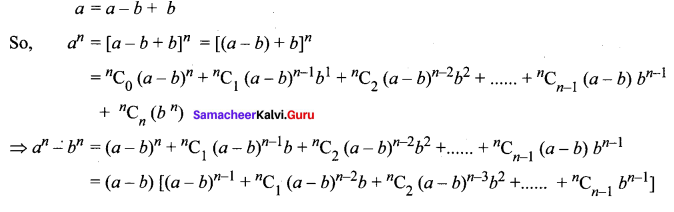= (a – b)[an integer]
⇒ an – bn is divisible by (a – b)

Chapter 5 Maths Class 11 Question 13.
In the binomial expansion of (a + b)an, the coefficients of the 4th and 13th terms are equal to each other, find n.
Solution:
In (a + b)n general term is tr + 1 = nCr an – r br
So, t4 = t3 + 1 = nC3 = nC12
⇒ n = 12 + 3 = 15
We are given that their coefficients are equal ⇒ nC3 = nC12 ⇒ n = 12 + 3 = 15
[nCx = nCy ⇒ x = y (or) x + y = n]

Class 11 Chapter 5 Maths Question 14.
If the binomial coefficients of three consecutive terms in the expansion of (a + x)n are in the ratio 1 : 7 : 42, then find n.
Solution:
In (a + x)n general term is tr + 1 = nCr
So, the coefficient of tr + 1 is nCr
We are given that the coefficients of three consecutive terms are in the ratio 1 : 7 : 42.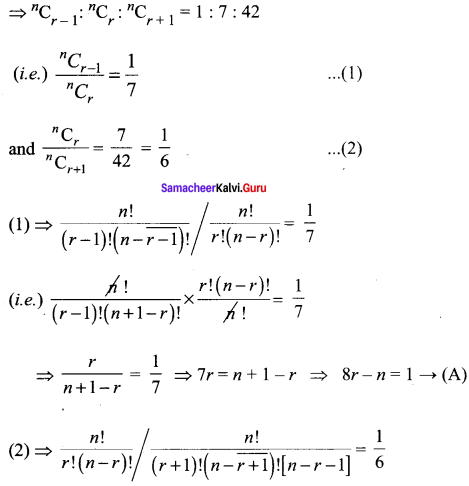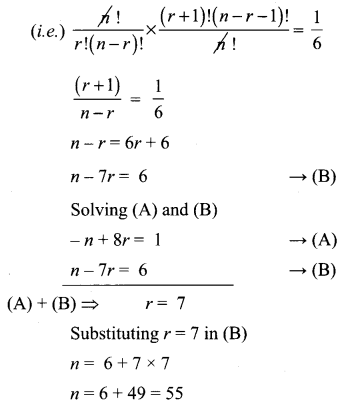Class 11 Maths Chapter 5 Question 15.
In the binomial coefficients of (1 + x)n, the coefficients of the 5th, 6th and 7 terms are in AP. Find all values of n.
Solution: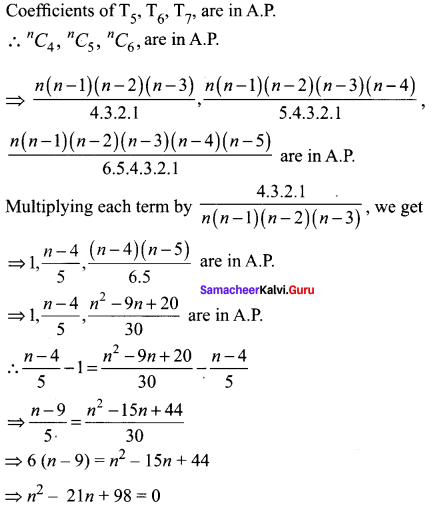⇒ (n – 1)(n – 14) = 0
∴ n = 7 , 14

Question 16.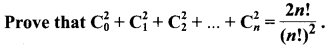Solution: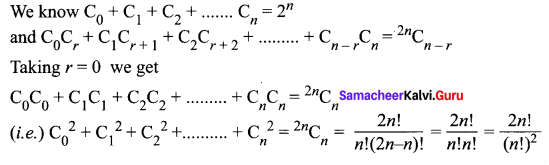### Samacheer Kalvi 11th Maths Solutions Chapter 5 Binomial Theorem, Sequences and Series Ex 5.1 Additional Questions Solved

Question 1.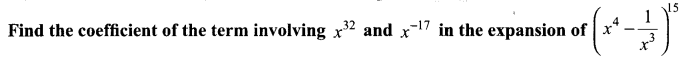Solution:
Let Tr + 1 be the term in which x32 and x-17 occurs,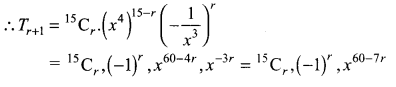(i) Since x32 occurs in this term
∴ Exponent of x = 32
⇒ 60 – 7r = 32 ⇒ 7r = 28
r = 28 ÷ 7 = 4
∴ Coefficient of the term containing x32 = 15C4 (-1)4 = 1365

(ii) Since x-17 occurs in this term .
∴ Exponent of x = -17
⇒ 60 – 7r = -17 ⇒ 7r = 77, ∴ r = 11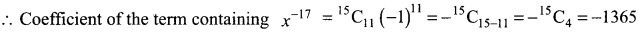Question 2.
Find a positive value of m for which the coefficient of x2 in the expansion of (1 + x)m is 6.
Solution: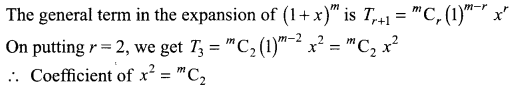Also, coefficient of x2 in the expansion of (1 + x)m is 6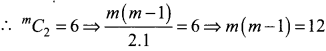⇒ m (m – 1) = 4.3 ⇒ m = 4

Question 3.
In the binomial expansion of (1 + a)m + n, prove that the coefficients of am and an are equal.
Solution: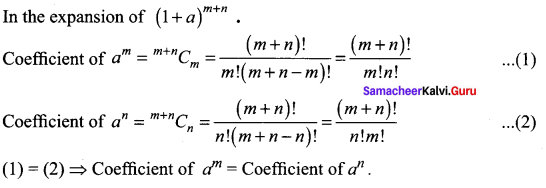Question 14.
The coefficient of (r – 1)th, rth, and (r + 1)th terms in the expansion of (x + 1)n are in the ratio 1 : 3 : 5. Find both n and r.
Solution:
We know that co-effcients of (r – 1)th, rth and (r + 1)th terms in the expansion of (x + 1)n are nCr – 2: nCr – 1 and nCr respectively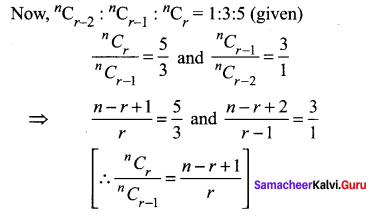⇒ 3n – 8r + 3 = 0 and n – 4r + 5 = 0
Solving these for n, r we get,
n = 7 and r = 3

Question 5.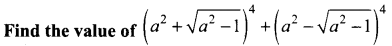Solution: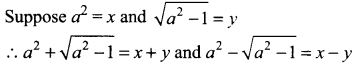Using binomial theorem,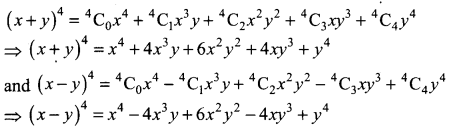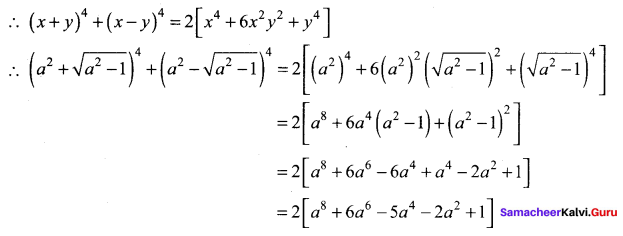Question 6.
Show that the coefficient of the middle term in the expansion of (1 + x)2n is equal to the sum of the coefficients of the two middle terms in the expansion of (1 + x)2n – 1.
Solution:
In the expansion of (1 + x)2n,
Number of terms = 2n + 1, which is odd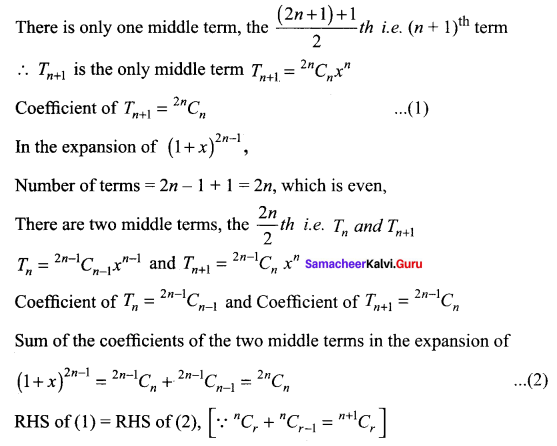Question 7.
If three consecutive coefficients in the expansion of (1 + x)n are in the ratio 6 : 33 : 110, find n.
Solution:
Let the consecutive coefficients nCr, nCr + 1 and nCr + 2 be the coefficients of Tr + 1, Tr + 2 and Tr + 3 then nCr : nCr + 1 : nCr + 2 = 6 : 33 : 110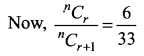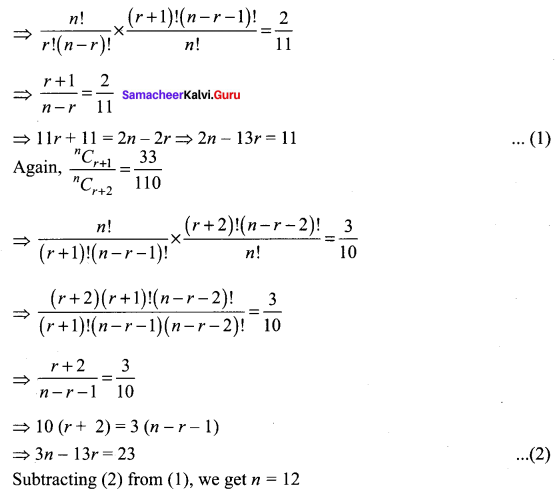Question 8.
If the sum of the coefficients in the expansion of (x + y)n is 4096. Then find the greatest coefficient in the expansion.
Solution:
Given that, Sum of the coefficients in the expansion of (x + y)n = 4096
nC0 + nC1 + nC2+…+ nCn = 4096
[∴ Sum of binomial coefficients in the expansion of (x + a)n is 2n]
⇒ 2n = 4096 = 212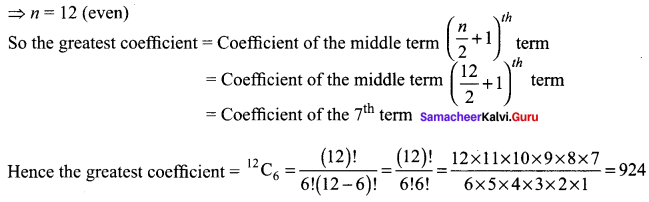Question 9.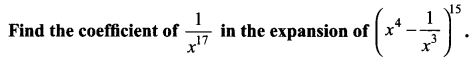Solution:
The general term in the expansion of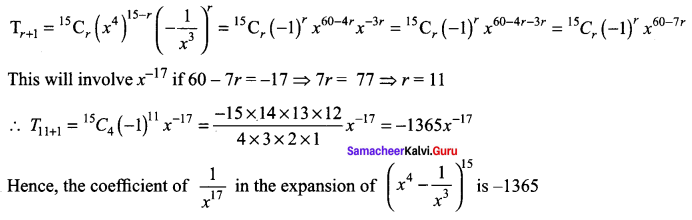Question 10.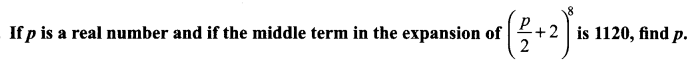Solution: